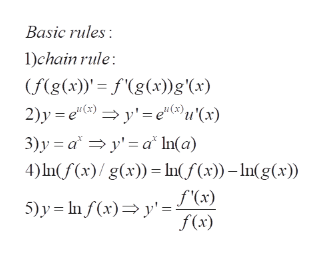# Find the derivative of the following equations: 1: y=e2x^2 - x +2x -3x2: y= ln(x3 / 2x2+3)

Question

Find the derivative of the following equations:

1: y=e2x^2 - x +2-3x

2: y= ln(x3 / 2x2+3)

check_circleExpert Solution
Step 1

To differentiate the given functions, by using basic rules of differentating standard functions (exponential, logarithmic)

Step 2

Rules (forumulae )...help_outlineImage TranscriptioncloseBasic rules 1)chain rule (f(g(x) fg(x))g'(x) 2)y e y'eu'(x) 3)y ay'a In(a) 4) nf(x)/ g(x))In(f(x))-In(g(x) f'(x) 5)y nf (x)y' f(x) fullscreen

### Want to see the full answer?

See Solution

#### Want to see this answer and more?

Solutions are written by subject experts who are available 24/7. Questions are typically answered within 1 hour*

See Solution
*Response times may vary by subject and question
Tagged in

### Calculus